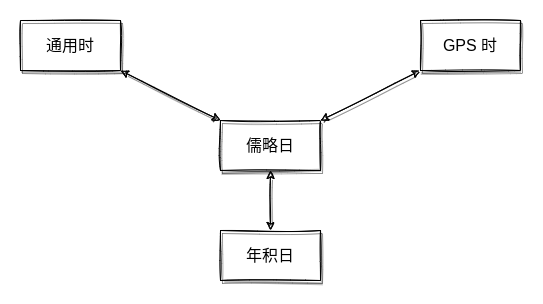# 空间大地测量时间系统

## 时间标示法

### GPS 时

GPS 系统内部所采用的时间系统是 GPS 时间，GPS 时以1980年1月6日子夜为起点，用周数（一个星期七天）和周内的秒数来表示。

## 各个时间系统的相互转换### 通用时与儒略日间的相互转换

API 函数：

CommonTimeToJulianDay( pct, pjd )


$$JD = INT[365.25y] + INT[30.6001(m+1)] + D + UT/24 + 1720981.5$$

• 如果 M≤2，则 y=Y-1，m=M+12；
• 如果 M>2，则 y=Y，m=M
• JD 为儒略日，Y 为年，M 为月，D 为日，UT 为世界时。

API 函数：

JulianDayToCommonTime( pjd, pct )


$$a = INT[JD + 0.5]$$

$$b = a + 1537$$

$$c = INT[(b-122.1)/365.25]$$

$$d = INT[365.25.c]$$

$$e = INT[(b-d)/30.6001]$$

$$D = b-d-INT[20.6001e]+FRAC[JD+0.5]$$

$$M = e-1-12*INT[e/14]$$

$$Y = c-4715-INT[(7+M)/10]$$

$$N = mod{INT[JD+0.5],7}$$

### 通用时与 GPS 时间间的相互转换

API 函数：

CommonTimeToGPSTime( pct, pgt )


• 第一步：计算 GPS 周
• 第二步：计算一周内的秒数

API 函数：

GPSTimeToCommonTime( pgt, pct )


• 第一步：由 GPS 时间转换到儒略日
• 第二步：由儒略日转换到通用时

### 儒略日与年积日之间的相互转换

API 函数：

JulianDayToDOY( pjd, pdoy )


• 第一步：计算出通用时
• 第二步：计算出当年1月1日的儒略日
• 第三步：两个儒略日求差加 1，得出年积日

API 函数：

DOYToJulianDay( pdoy, pjd )


• 第一步：计算出当年1月1日的儒略日 JD1
• 第二步：年积日加 JD1 减 1 得儒略日

### GPS 时与年积日间的相互转换

GPS 时转换到年积日

API 函数：

GPSTimeToDOY ( pgt, pdoy )


API 函数：

DOYToGPSTime (pdoy, pgt )